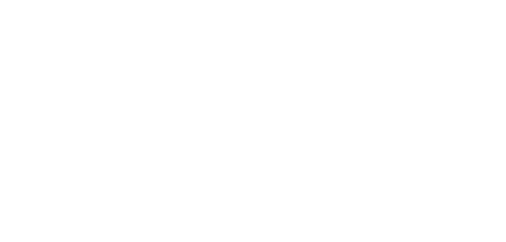# function and their types in C++

## Function in C++

In simple way, The function is a set of statements.

let’s explore it,

It is not easy to manage many statements together in a single program, so we divided these into small pieces or modules. These set of statements (which contain the code to perform a particular task) are called from the other part of the program. These modules are called function.

## Function declaration in C++

Declaring a function in name in C++ are called function-Declaration.

Here is the syntax of Declaring a function in C++,

``return-type function-name(parameter-list);``

Here the function-name is an identifier i.e. any appropriate name to the function while parameter-list are data types.

## C++ Function definition

This is the main body of the function in which the code is written to perform a task, we call the definition of any function to the other part of the program. We can also call a function multiple times in the program as per our requirement.

Remember here, we can not call a function without its function definition if we do this a error will be occur in the program at run-time (not compile-time) known as Linker error.

## use of function in C++

• The program can be easily read.
• Easy debugging of program.
• There is no need to write an extra statement to perform the same task, ie these can be reuse to perform the same task. which saves time.
• Because using the function does not have to be written repeatedly for the same task, so the size of the program is reduced which increases the speed of the program.

## Type of function in C++

• inbuilt functions
• user-defined functions

### inbuilt Functions in C++

such functions are already defined in the library of C ++, we can use them by including them in the header file program! The use of inbuilt function in a program makes the program easy, meaning that we do not need to code separately for any task. For example-

system(“pause”) used to hold the  output on the screen.

### user defined function in C++

These are the function that programmers create themselves to perform a task. Their definition consists of a code or set of statement to perform a task. In C++ we can declare the function in three ways which are as follows-

#### no argument and no return type function

when we declare the function without parameter-list, these are also called void type functions, because, in the function declaration, void declare inside the bracket in the function header whoever does not return any value.

syntax

``void function-name(void);``

Here is the program , where void sum(void); is function type

``````#include<iostream>
using namespace std;
void sum(void); // function declaration

int main()
{
sum(); //function calling
return 0;
}

void sum() // function definition
{
int num1,num2,total; // variable declaration

cout<<"Enter two number: ";
cin>>num1>>num2;

total=num1+num2;
cout<<"Total of these Number: "<<total;
}``````

OUTPUT

``````Enter two number: 5 6
Total of these number: 11``````

#### argument but no return type function

This type of functions defines some parameters (data type) inside the bracket in their header while making the function declaration, because their return-type is void type data-type so they do not return any value.

syntax

``void function-name(data-type);``

Here is the program,

``````#include<iostream>
using namespace std;
void sum(int,int);          // function declaration

int main()
{
int num1,num2; // variable declaration

cout<<"Enter two number: ";
cin>>num1>>num2;

sum(num1,num2); // function calling
return 0;
}

void sum(int x,int y) // function definition
{
int total;
total=x+y;

cout<<"Total of these Number: "<<total;
}``````

OUTPUT

``````Enter two number: 7 8
Total of these number: 15``````

#### argument and return type function

In this type of function declaration, we first declare return data type (except for `void`), then second function-name and in the last we declare some parameters in parenthesis bracket. These values return. which can be of type `int, char` or `float` These functions are not void types.

syntax

``data-type function-name(data-type);``

Here is the program,

``````#include<iostream>
using namespace std;
int sum(int,int); // protoype declaration

int main()
{

int num1,num2,total; // variable declaration
cout<<"Enter two number: ";
cin>>num1>>num2;

total=sum(num1,num2); // function calling

cout<<"Total of these Number: "<<total;
return 0;
}

int sum(int x,int y) // function definition
{
return x+y;
}``````

OUTPUT

``````Enter two number: 6 8
Total of these number: 14``````

## actual parameter in C++

The parameters declared in the calling function-header  (inside the parenthesis bracket) are called actual parameters.

syntax

``function-name(parameter-list);``

Example

``calculate(a,b);``

a and b are called actual parameters.

see the diagram below,## formal parameter in C++

Parameters defined in the function definition-header(inside the p) are called formal parameter.

syntax

``````function-name(parameter-list)
{
Body of function;
}``````

Example

``````calculate (int x , int y)
{
body of function;
}``````

Here x and y are formal parameters.

Let’s try with an example

``````#include<iostream.h>
using namespace std;
void calculate(int,int); // function declaration

int main()
{

int a,b; // variable declaration

cout<<"Enter two number: ";
cin>>a>>b;

calculate(a,b); // function calling (actual parameter)
return 0;
}

void calculate (int x,int y) // function definition (formal parameter)
{
cout<<"\nmultiplication: "<<x*y;
cout<<"\nsubstraction : "<<x-y;
}``````

OUTPUT

``````Enter two number: 4 5
multiplication: 20

substraction : -1``````

Things to know

Function is a method that provides the facility to reuse the code to perform the same task, whereas code from control statement is written for a task. Therefore it is important to understand the control statement closely. Data Structure is based on control statement, array and pointer.

Related exercise